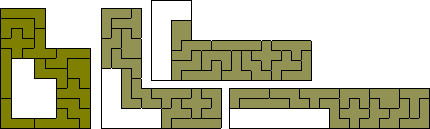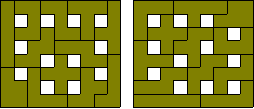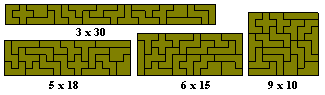Pentomino Patterns and Problems

For a specific problem of tiling rectangles with extra copies of one of the pieces see Enhanced Sets of PentominoesA large number of problems have been considered with pentominoes in the last 40 years and many of these are discussed on the sites listed above and here we shall just include a few which are not discussed elsewhere.

1. The 15-60 problem

Here the problem is to use 3 pentominoes to form a 15 square region and then add the remaining 9 pieces to complete a duplicated copy of the original area. Four solutions are shown below.In the solution at the right (from Mike Reid) the 15 squre region is produced twice and so could be said to solve a 15-15-60 problem.2. The similar hole problem.

This problem was originally proposed by Jean Meeus in 1974 and involves creating a figure with an internal hole which is the same shape. One example of this is an 8x10 rectangle with a 4x5 hole and four examples are shown below.Meeus has shown that the four positions of the 4x5 rectangle are the only possible and that the solution to symmetrical case (second from the left) is unique. Another example of this problem is to create quadruplications of the tetrominoes with a hole in the shape of the tetromino - all five are possible.

Mike Reid has found another example shown at the left below. This is a 4-fold copy of the P-pentomino with a 2-fold copy removed. The three figures on the right are similar but the 'holes' are at the edge and thus these do not provide valid solutions.We can also look for multiple congruent similar hole shapes.3. The maximum hole problem.

What is the maximum number of unit square holes which can exist without touching each other in a pentomino construction? It is not too hard to show that 14 is impossible so the problem is to find 13-hole solutions. Three are included here.The solutions above shown only two different patterns of holes but others are possible including the three shown below.If alternatively we look to find the maximum nuber of holes in a rectangular region then we get 12 holes in the 8x9 rectangle where two solutions, one with reflectional and the other rotational symmetry are shown.4. The Triplication.Problem

If we take 9 of the pentominoes we can make a threefold replica of each of the pentominoes. Not all sets of nine pieces can be used for each triplication. There are just 15 solutions for the X pentomino if the X is omitted.A full analysis of this problem is here.

We can also use 9 sets of pentominoes to form simultaneous triplications of all 12 pentominoes. One solution is shown below. This is not a particularly nice solution since the same pentomino is used more than once in some of the triplications (see the three I's, 2 T's and 2 Y's in the triplicated Y). Can anyone find a solution where each triplication contains no duplication of pieces?Aad van de Wetering has now found a solution with no duplication of pieces. This is one of 69,153 solutions which are possible if the pentomino being triplicated is not used in the triplication. If you would like to find other solutions then the tables giving the various combinations of pieces which can be used for each triplication are here.One-sided Pentominoes

If we consider mirror image pairs as distinct then there are 18 pentominoes. This set would cover an area of 90 unit squares and can form 3 x 30, 5 x 18, 6 x 15 and 9 x 10 rectangles. The 3x30 rectangle has 46 solutions.One problem for the one-sided pentominoes is to create pairs of triplications. In particular to triplicate a mirror image pair. A number of examples can be seen below (all but the solution at the top left are by Roel Huisman).Other variations of this would be to form two congruent shapes such as the examples below.Three congruent shapes can be made from this set as in the example below.Many other symmetrical patterns are also possible with this set. The construction at the right is a triplication of a dekomino as is the 3 x 30 rectangle.We can also consider the maximum hole problems for this set. The best known solutions are 21 squares (23 is not possible) for the maximum and 17 in a 9x12 rectangle.A number of similar hole constructions are also possible with this set. In particular we can make four-fold copies of each of the hexominoes with a hole in the shape of an hexomino.This set can also be used to form simultaneous pairs of triplications.